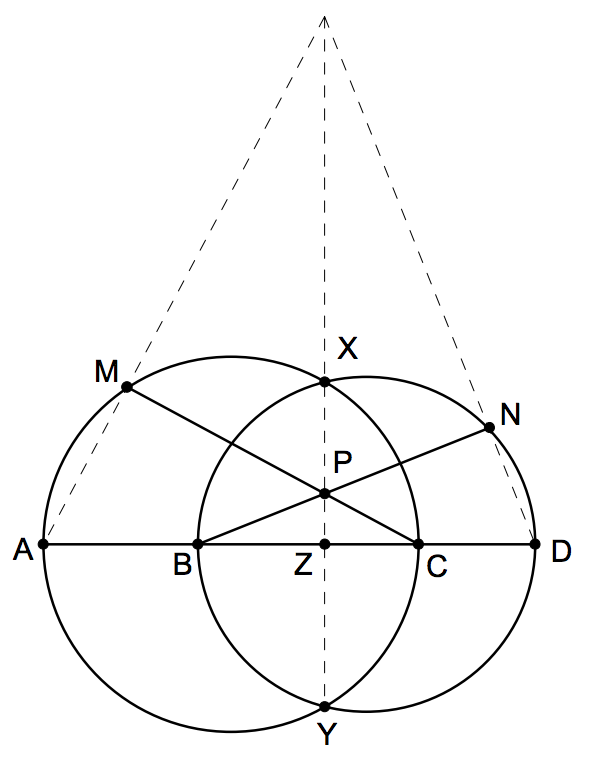#### PlaneGeometry IMO

###### back to index

Let $ABCD$ be a cyclic quadrilateral. Let $P, Q, R$ be the feet of the perpendiculars from $D$ to the lines $BC, CA$ and $AB$ respectively. Show that $PQ = QR$ iff the bisectors of $\angle ABC$ and $\angle ADC$ meet on $AC$.

Let $ABCD$ be a convex quadrilateral for which $AC = BD$. Equilateral triangles are constructed on the sides of the quadrilateral and pointing outward. Let $O_1, O_2, O_3, O_4$ be the centres of the triangles constructed on $AB, BC, CD,$ and $DA$ respectively. Prove that lines $O_1O_3$ and $O_2O_4$ are perpendicular.

Given an acute $\triangle{ABC}$ with side length $BC > CA$.

Let $A$, $B$, $C$ and $D$ be four distinct points on a line, in that order. The circles with diameters $AC$ and $BD$ intersect at the points $X$ and $Y$. The line $XY$ meets $BC$ at the point $Z$. Let $P$ be a point on the line $XY$ different from $Z$. The line $CP$ intersects the circle with diameter $AC$ at the points $C$ and $M$, and the line $BP$ intersects the circle with diameter $BD$ at the points $B$ and $N$. Prove that the lines $AM$, $DN$ and $XY$ are concurrent(Weitzenbock's Inequality) Let $a, b, c$, and $S$ be a triangle's three sides' lengths and its area, respectively. Show that $$a^2 + b^2 + c^2 \ge 4\sqrt{3}\cdot S$$

The diagonals $AC$ and $CD$ of the regular hexagon $ABCDEF$ are divided by inner points $M$ and $N$ such that $AM:AC = CN:CE=r$. Determine $r$ if $B, M,$ and $N$ are collinear.

Prove that there is one and only one triangle whose side lengths are consecutive integers, and one of whose angles is twice as large as another.

Let $\Gamma$ be the circumcircle of acute triangle $ABC$. Points $D$ and $E$ are on segments $AB$ and $AC$ respectively such that $AD = AE$. The perpendicular bisectors of $BD$ and $CE$ intersect minor arcs $AB$ and $AC$ of $\Gamma$ at points $F$ and $G$ respectively. Prove that lines $DE$ and $FG$ are either parallel or they are the same line.

A convex quadrilateral $ABCD$ satisfies $AB\cdot CD=BC \cdot DA.$ Point $X$ lies inside $ABCD$ so that $\angle XAB = \angle XCD$ and $\angle XBC = \angle XDA.$ Prove that $\angle BXA + \angle DXC = 180^{\circ}$ .